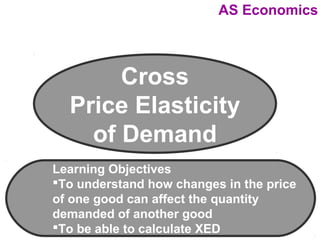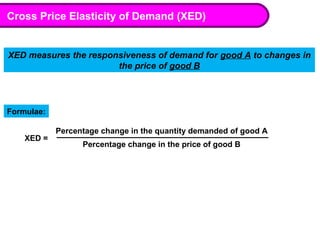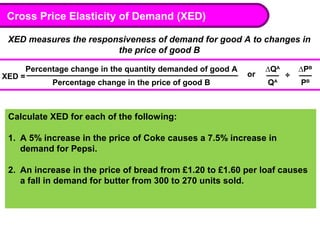Se está descargando tu SlideShare. ×

# Cross price elasticity of demandCargando en…3
×

1 de 6
1 de 6

# Cross price elasticity of demand

### Cross price elasticity of demand

1. 1. AS Economics Cross Price Elasticity of Demand Learning Objectives To understand how changes in the price of one good can affect the quantity demanded of another good To be able to calculate XED
2. 2. Cross Price Elasticity of Demand (XED) XED measures the responsiveness of demand for good A to changes in the price of good B Formulae: XED = Percentage change in the quantity demanded of good A Percentage change in the price of good B
3. 3. Cross Price Elasticity of Demand (XED) XED measures the responsiveness of demand for good A to changes in the price of good B XED = Percentage change in the quantity demanded of good A Percentage change in the price of good B or ∆QA Q A ÷ ∆PB PB Calculate XED for each of the following: 1. A 5% increase in the price of Coke causes a 7.5% increase in demand for Pepsi. 2. An increase in the price of bread from £1.20 to £1.60 per loaf causes a fall in demand for butter from 300 to 270 units sold.
4. 4. Cross Price Elasticity of Demand (XED) XED measures the responsiveness of demand for good A to changes in the price of good B XED = Percentage change in the quantity demanded of good A Percentage change in the price of good B or ∆QA Q A ÷ ∆PB PB Interpreting XED results: Positive XED: Substitute goods – as price of good B rises, quantity demanded of good A rises E.g. Coke and Pepsi Negative XED: Complementary goods – as price of good B rises, quantity demanded of good A falls. E.g. bread and butter
5. 5. Cross Price Elasticity of Demand (XED) XED measures the responsiveness of demand for good A to changes in the price of good B XED = Percentage change in the quantity demanded of good A Percentage change in the price of good B or ∆QA Q A ÷ ∆PB PB For each of the following calculate the XED and identify if the goods are substitutes or complements: 1. The price of petrol rises by 20% causing demand for cars to fall 5% 2. Demand for margarine falls 10% following a 20% cut in butter prices 3. A 20% rise in the price of toothpaste, a 15% fall in demand for cats. Fish and chips are complementary goods with a cross elasticity of demand equal to -8. Currently 50 000 fish are sold. Calculate the new demand for fish following a 2% cut in the price of chips.
6. 6. Cross Price Elasticity of Demand (XED) XED measures the responsiveness of demand for good A to changes in the price of good B XED = Percentage change in the quantity demanded of good A Percentage change in the price of good B or ∆QA Q A ÷ ∆PB PB For each of the following calculate the XED and identify if the goods are substitutes or complements: 1. The price of petrol rises by 20% causing demand for cars to fall 5% 2. Demand for margarine falls 10% following a 20% cut in butter prices 3. A 20% rise in the price of toothpaste, a 15% fall in demand for cats. Fish and chips are complementary goods with a cross elasticity of demand equal to -8. Currently 50 000 fish are sold. Calculate the new demand for fish following a 2% cut in the price of chips.